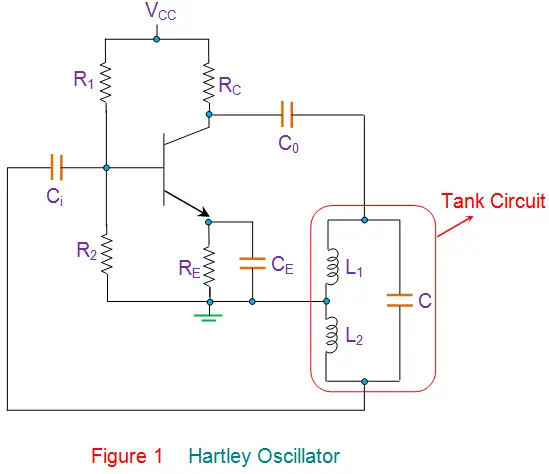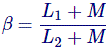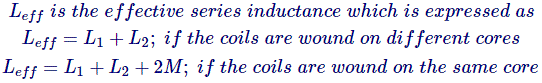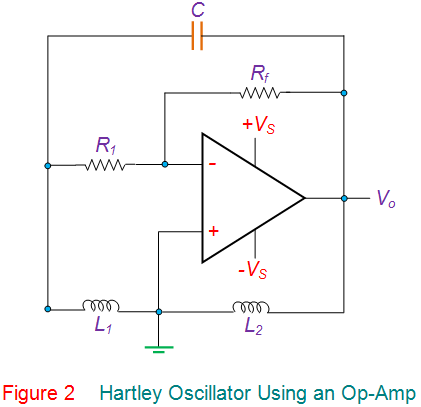# Hartley Oscillator: What is it? (Frequency And Circuit)## What is a Hartley Oscillator?

A Hartley Oscillator (or RF oscillator) is a type of harmonic oscillator. The oscillation frequency for a Hartley Oscillator is determined by an LC oscillator (i.e. a circuit consisting of capacitors and inductors). Hartley oscillators are typically tuned to produce waves in the radiofrequency band (which is why they are also known as RF oscillators).

Hartley Oscillators were invented in 1915 by American engineer Ralph Hartley.

The distinguishing feature of a Hartley oscillator is that the tuning circuit consists of a single capacitor in parallel with two inductors in series (or a single tapped inductor), and the feedback signal needed for oscillation is taken from the center connection of the two inductors.

A circuit diagram for a Hartley Oscillator is shown below in Figure 1:Here the RC is the collector resistor while the emitter resistor RE forms the stabilizing network. Further the resistors R1 and R2 form the voltage divider bias network for the transistor in common-emitter CE configuration.

Next, the capacitors Ci and Co are the input and output decoupling capacitors while the emitter capacitor CE is the bypass capacitor used to bypass the amplified AC signals. All these components are identical to those present in a common-emitter amplifier which is biased using a voltage divider network.

However, Figure 1 also shows one more set of components viz., the inductors L1 and L2, and the capacitor C which form the tank circuit (shown in the red enclosure).

On switching ON the power supply, the transistor starts to conduct, leading to an increase in the collector current, IC which charges the capacitor C.

On acquiring the maximum charge feasible, C starts to discharge via the inductors L1 and L2. These charging and discharging cycles result in the damped oscillations in the tank circuit.

The oscillation current in the tank circuit produces an AC voltage across the inductors L1 and L2 which are out of phase by 180o as their point of contact are grounded.

Further from the figure, it is evident that the output of the amplifier is applied across the inductor L1 while the feedback voltage drawn across L2 is applied to the base of the transistor.

Thus one can conclude that the amplifier’s output is in-phase with the tank circuit’s voltage and supplies back the energy lost by it while the energy fed back to the amplifier circuit will be out-of-phase by 180o.

The feedback voltage which is already 180o out-of-phase with the transistor, is provided by an additional 180o phase-shift due to the transistor action.

Hence the signal which appears at the transistor’s output will be amplified and will have a net phase-shift of 360o.

At this state, if one makes the gain of the circuit to be slightly greater than the feedback ratio given by(if the coils are wound on the same core with M indicating the mutual inductance)
then the circuit generates the oscillator that can be sustained by maintaining the circuit’s gain to be equal to that of the feedback ratio.

This causes the circuit in Figure 1 to act as an oscillator as it would then satisfy both the conditions of the Barkhausen criteria.

The frequency of such an oscillator is given asWhere,Hartley oscillators are available in many different configurations including series-or shunt-fed, common-emitter or common-base configured, and BJT (Bipolar Junction Transistor) or FET (Field Effect Transistor) amplifier based.

Further, it is to be noted that the transistor-based amplifier section of Figure 1 can even be replaced by an amplifier of any other kind like that of an inverting amplifier formed by an Op-Amp as shown by Figure 2.

The working of this kind of oscillator is similar to that of the one shown earlier. However, here, the oscillator’s gain can be individually adjusted using the feedback resistor Rf due to the fact that the gain of the inverting amplifier is given as -Rf / R1.

From this, it can be noted that, in this case, the gain of the circuit is less dependent on the circuit elements of the tank circuit.

This increases the stability of the oscillator in terms of its frequency.Hartley Oscillators are advantageous as they are easy-tunable circuits with very few components including a capacitor and either two inductors or a tapped coil.

This results in a constant amplitude output throughout its wide operational frequency r, ranging from 20 kHz to 30 MHz. However, this kind of oscillator is not suitable for low frequency as it would result in a large-sized inductor which makes the circuit bulky.

Further, a Hartley Oscillator’s output has high harmonics content in it and hence does not suit the applications that require pure sine wave.

Want To Learn Faster? 🎓
Get electrical articles delivered to your inbox every week.
No credit card required—it’s 100% free.